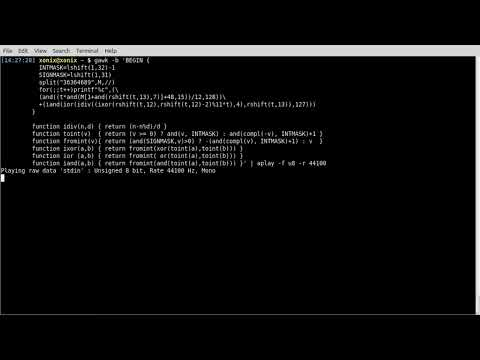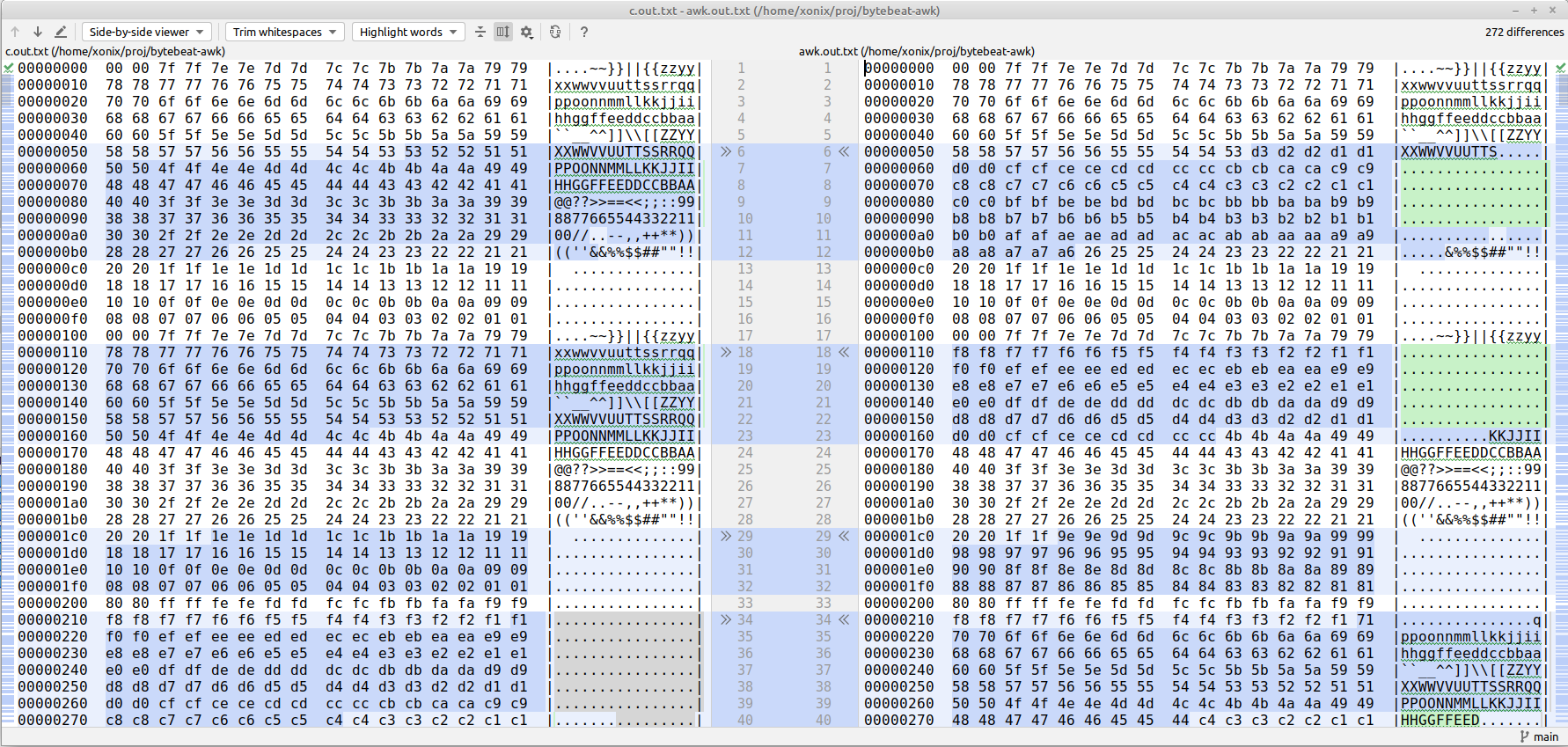# Volodymyr Gubarkov# Bytebeating with GAWK

April 2023

Not long ago I came across this blog post. It describes a simple awk script that is able to generate harmonious audio sounds.

I immediately recalled the bytebeat – a technique of generating interesting sound effects and even the whole melodies in couple lines of C code.

I thought – would it be possible (just for fun, of course) to convert some of bytebeats from C to GAWK?

Why AWK? Because I’m a huge fan of it. Why particularly GNU AWK? Because only GNU AWK variant has built-in bitwise functions.

Here is the result of my effort (link to YouTube, please turn down the volume a bit before you click, just in case):Below I would like to share some of the learnings I have gained along the way.

The idea of bytebeat is pretty simple. It exploits the old Unix principle that everything is a file. So generating music this way is as easy as running:

``````./prog > /dev/dsp
``````

Where `./prog` is a (compiled C, for example) program, that outputs stream of bytes, and `/dev/dsp` is a “virtual file” representing the audio input device.

Unfortunately, modern Linuxes don’t expose audio devices in form of a file.

However, nowadays, you have plenty of ways to achieve the similar result. For example, in Linux you have `aplay` command – “command-line sound player for ALSA soundcard driver”. So, the same command looks like:

``````./prog | aplay
``````

Let’s take a look at a simple bytebeat:

``````// file: a1.c
main(t) {
for(t=0;;t++)putchar(
t*((t>>12|t>>8)&63&t>>4) // <-- formula that defines the melody
);}
``````

Now, to make it play you need:

``````\$ cc -w a1.c -o a1
\$ ./a1 | aplay -f u8
Playing raw data 'stdin' : Unsigned 8 bit, Rate 8000 Hz, Mono
``````

The conversion to GAWK (using the bitwise functions) is pretty straightforward:

``````# file: a1.awk
BEGIN { for(;;t++)
printf"%c",t*and(or(rshift(t,12),rshift(t,8)),63,rshift(t,4))
}
``````

However, running it, you can immediately notice, that the sound it produces is not the same as of the C source:

``````\$ gawk -f a1.awk | aplay -f u8
Playing raw data 'stdin' : Unsigned 8 bit, Rate 8000 Hz, Mono
``````

What’s the problem?

Obviously, the GAWK variant generates different stream of bytes than the C.

Not understanding what’s going on, I decided to start from the simplest formula possible: `t`. I also decided to generate a fixed number of bytes (`10000`) by both programs and try to compare the outputs.

``````// file tmp.c
main(t) {
for(t=0;t<10000;t++)putchar(
t
);}
``````
``````# file: tmp.awk
BEGIN { for(;t<10000;t++)
printf"%c",t
}
``````

Generating outputs:

``````\$ cc -w tmp.c -o tmp
\$ ./tmp > tmp.c.out
\$ gawk -f tmp.awk > tmp.awk.out
``````

The first obvious thing – let’s check the length of both files:

``````\$ ls -l tmp.*.out
-rw-rw-r-- 1 xonix xonix 27824 Apr 18 20:07 tmp.awk.out
-rw-rw-r-- 1 xonix xonix 10000 Apr 18 20:07 tmp.c.out
``````

Here is it! The C output is 10000 bytes long as expected, but GAWK generates a longer file.

Let’s look at putchar C standard function. According to the documentation, it writes a character (represented by `int`) to stdout by converting it to `unsigned char` just before being written. If we take a closer look at C standard types we find that `int` is (signed) 4 bytes long, and `unsigned char` is unsigned 1 byte long.

So, eventually, what `putchar` does is it takes the `int` argument and only uses the lowest byte of it. The rest of the argument value is just discarded.

Let’s make a small experiment.

C:

``````\$ echo 'main(){putchar(9786);}' | tcc -w -run -
:
``````

(here I use tcc by genius Fabrice Bellard for convenience).

GAWK:

``````\$ gawk 'BEGIN { printf"%c",9786 }'
☺
``````

Long story short, it appears that GAWK by default operates on unicode characters, not bytes. But it has `-b` option that allows to work with strings as with single-byte characters.

``````\$ gawk -b 'BEGIN { printf"%c\n",9786 }'       # now the output matches C!
:
\$ gawk -b -f tmp.awk > tmp.awk.out
\$ ls -l tmp.*.out
-rw-rw-r-- 1 xonix xonix 10000 Apr 18 21:33 tmp.awk.out
-rw-rw-r-- 1 xonix xonix 10000 Apr 18 20:07 tmp.c.out
``````

Excellent! Now the sound plays as intended:

``````\$ gawk -b -f a1.awk | aplay -f u8
Playing raw data 'stdin' : Unsigned 8 bit, Rate 8000 Hz, Mono
``````

Using the same conversion technique I was able to convert a couple more bytebeats from C to GAWK:

``````gawk -b 'BEGIN { for(;;t++)printf"%c",t*and(or(rshift(t,12),rshift(t,8)),63,rshift(t,4)) }' | aplay -f u8

gawk -b 'BEGIN { for(;;t++)printf"%c",and(t,t%255)-and(t*3,rshift(t,13),rshift(t,6)) }' | aplay -f u8

gawk -b 'BEGIN { for(;;t++)printf"%c",or(and(t*5,rshift(t,7)),and(t*3,rshift(t*4,10))) }' | aplay -f u8

gawk -b 'BEGIN { for(;;t++)printf"%c",or(t,or(rshift(t,9),rshift(t,7)))*and(t,or(rshift(t,11),rshift(t,9)))}' | aplay -f u8
``````

If you are on Linux, you can try each of them straight in the command line.

During the conversion it was crucial to understand the operators precedence in C. Without paying close attention to this you risk to put `()` in wrong order, not equivalent to the logic of the C source.

Finally, I wanted to try something more complex. I chose this bytebeat by ryg that resembles a melody:

``````main(t) {
for(;;t++)putchar(
((t*("36364689"[t>>13&7]&15))/12&128)
+(((((t>>12)^(t>>12)-2)%11*t)/4|t>>13)&127)
);}
``````

This is where GAWK started to fail miserably. The reason for this was that C allows binary operations on negative numbers. After all, it’s a binary representation of a number that matters, so you are allowed to do it, provided that you understand what you are doing. However, GAWK chose to explicitly disallow. It appears, the bytebeat above uses heavily binary operation on negatives ☹.

Let me show you the problem.

C:

``````\$ echo
'main(){printf("%d\n",5^2);printf("%d\n",-5^2);printf("%d\n",5^-2);printf("%d\n",-5^-2);}'
| tcc -w -run -
7
-7
-5
5
``````

JavaScript (node + browsers):

``````\$ node -e
'console.log(5^2);console.log(-5^2);console.log(5^-2);console.log(-5^-2);'
7
-7
-5
5
``````

Python:

``````\$ python3 -c 'print(5^2);print(-5^2);print(5^-2);print(-5^-2)'
7
-7
-5
5
``````

Gawk:

``````\$ gawk 'BEGIN { print xor(5,2);print xor(-5,2);print xor(5,-2);print xor(-5,-2); }'
7
gawk: cmd. line:1: fatal: xor: argument 2 negative value -5 is not allowed
``````

I wondered – is it possible to emulate in GAWK the bitwise logic on negative numbers according to C and other languages?

I knew that GAWK internally works with numbers using (64 bits) double-precision floating points (`double` in C). If we take a look at a binary representation of doubles we’ll find that they use 53 bits of precision which is far more than 32 bits needed to represent `int`. So it should be absolutely possible to emulate binary operations on (signed) `int`-s on top of `double` representation.

First step here is to understand how signed (or, particularly, negative) integers are represented in binary form. It turns out, the Two’s complement mechanism is the key here.

This gives a clue to a possible solution. We need to convert a GAWK number (`double`) into some other (positive) `double` representation that will “play a role” of (signed) `int`, eligible for GAWK binary functions. We will also need the reverse conversion to convert “`int`-like” `double`-s back to “ordinary” `double`-s.

This is exactly how I coded it (functions `toint` and `fromint`) according to the Two’s complement algorithm mentioned above. Luckily GAWK provides the `compl()` function, needed for bitwise complement, making the solution possible.

Overall, I would prefer if GAWK used the approach taken by JavaScript:

JavaScript stores numbers as 64 bits floating point numbers, but all bitwise operations are performed on 32 bits binary numbers. Before a bitwise operation is performed, JavaScript converts numbers to 32 bits signed integers. After the bitwise operation is performed, the result is converted back to 64 bits JavaScript numbers.

I also want to mention some other techniques I’ve used for debugging the converted result in attempt to make it produce the same output (and therefore the sound) as the source.

I used the same technique of generating the fixed count of bytes and comparing the outputs from the source and the converted bytebeats. To localize the problem I debugged the output on smaller parts of the formula, making it converge piece-by-piece. That is, instead of trying to fight the whole formula `((t*("36364689"[t>>13&7]&15))/12&128)+(((((t>>12)^(t>>12)-2)%11*t)/4|t>>13)&127)` I took just `t>>13&7` and made it convert correctly, then `"36364689"[t>>13&7]`, and so on.

It’s not easy to compare binary outputs directly, so I used the `hexdump` utility to turn binary into human-readable text. Afterward I’ve used file comparison in my IDE to spot the difference:For this purpose I’ve used the small script to generate the files:

``````set -e

X=a3

tcc -w -run \$X.c > c.out||true ; hexdump -C c.out   > c.out.txt
gawk -f \$X.awk -b > awk.out    ; hexdump -C awk.out > awk.out.txt
``````

The other learning was that I needed to add `-C` flag to `hexdump`, otherwise due to endiannes inconsistency it was swapping the bytes in the text output.

Also, it was interesting to measure the generation speed of C vs GAWK. For this purpose I’ve used the `pv` utility:

``````\$ ./a3 | pv > /dev/null
874MiB 0:00:14 [61.6MiB/s]

\$ gawk -f a3final.awk -b | pv >/dev/null
5.94MiB 0:00:21 [ 293KiB/s]
``````

So according to this C is roughly 210x times faster than GAWK `¯\_(ツ)_/¯`

Let’s check what speed is enough for playback:

``````\$ ./a3 | pv | aplay -f u8 -r 44100
Playing raw data 'stdin' : Unsigned 8 bit, Rate 44100 Hz, Mono
784KiB 0:00:16 [46.5KiB/s]
``````

So GAWK still has 6x margin.

## Conclusion

All in all, it was a pretty amusing adventure.

I enjoyed the wonderful bytebeats - tiny C programs producing sounds and even melodies.

I learned some interesting details on binary arithmetic and how it’s done under the hood of the computer.

I can’t but recommend this exercise to other programmers. Try converting some bytebeats from C to your favorite programming language. Probably, you’ll like it as much as I did!

If you noticed a typo or have other feedback, please email me at xonixx@gmail.com